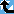# Fibonacci Number

The Prime Pages keeps a list of the 5000 largest known primes, plus a few each of certain selected archivable forms and classes. These forms are defined in this collection's home page.

A Fibonacci prime, as you should easily guess, is a Fibonacci number that is prime. Recall that the Fibonacci numbers can be defined as follows: u1 = u2 = 1 and un+1 = un + un-1 (n > 2).

It is easy to show that un divides unm (see primitive part of a Fibonacci number), so for un to be a prime, the subscript must either be 4 (because u2=1) or a prime. This however is not sufficient!

The list of known Fibonacci primes begins un with

n = 3, 4, 5, 7, 11, 13, 17, 23, 29, 43, 47, 83, 131, 137, 359, 431, 433, 449, 509, 569, 571, 2971, 4723, 5387, 9311, 9677, 14431, 25561, 30757, 35999, 37511, 50833 and 81839.
They are probable-prime for n = 104911 [Bouk de Water], 130021 [D. Fox], 148091 [T. D. Noe] and 201107, 397379, 433781 [H. Lifchitz]

###Record Primes of this Type

rankprime digitswhowhencomment
1U(201107) 42029 E11 Sep 2023 Fibonacci number, ECPP
2U(148091) 30949 x49 Sep 2021 Fibonacci number, ECPP
3U(130021) 27173 x48 May 2021 Fibonacci number, ECPP
4U(104911) 21925 c82 Oct 2015 Fibonacci number, ECPP
5U(81839) 17103 p54 Apr 2001 Fibonacci number
6U(50833) 10624 CH4 Oct 2005 Fibonacci number
7U(37511) 7839 x13 Jun 2005 Fibonacci number
8U(35999) 7523 p54 Jul 2001 Fibonacci number, cyclotomy
9U(30757) 6428 p54 Jul 2001 Fibonacci number, cyclotomy
10U(25561) 5342 p54 Jul 2001 Fibonacci number
11U(14431) 3016 p54 Apr 2001 Fibonacci number
12U(9677) 2023 c2 Nov 2000 Fibonacci number, ECPP
13U(9311) 1946 DK Mar 1995 Fibonacci number
14U(5387) 1126 WM Jan 1991 Fibonacci number

###References

BMS1988
J. Brillhart, P. Montgomery and R. Silverman, "Tables of Fibonacci and Lucas factorizations," Math. Comp., 50 (1988) 251--260.  MR 89h:11002
Brillhart1999
J. Brillhart, "Note on Fibonacci primality testing," Fibonacci Quart., 36:3 (1998) 222--228.  MR1627388
DK99
H. Dubner and W. Keller, "New Fibonacci and Lucas primes," Math. Comp., 68:225 (1999) 417--427, S1--S12.  MR 99c:11008 [Probable primality of F, L, F* and L* tested for n up to 50000, 50000, 20000, and 15000, respectively. Many new primes and algebraic factorizations found.]
LRS1999
Leyendekkers, J. V., Rybak, J. M. and Shannon, A. G., "An analysis of Mersenne-Fibonacci and Mersenne-Lucas primes," Notes Number Theory Discrete Math., 5:1 (1999) 1--26.  MR 1738744